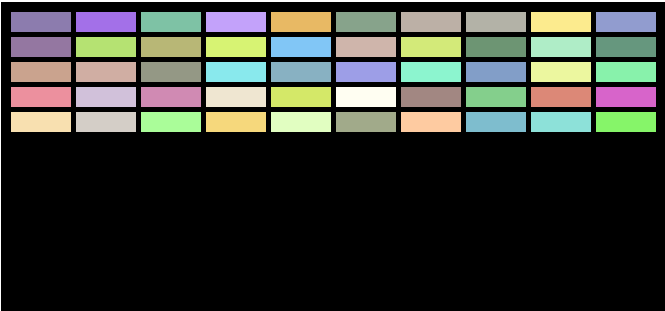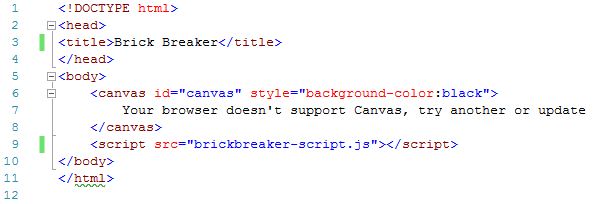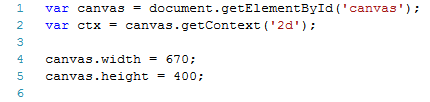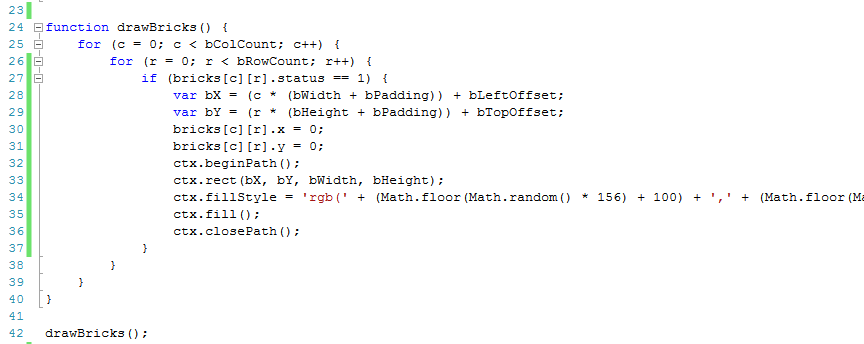# Canvas Basics – 09 More On ArraysThis is the ninth post in this series looking at the <canvas> element in HTML5.

Each post will give a new element of snippet of code that can be put together to create your own games.

So far we have covered the main elements needed to create a basic Pong-style tennis game.  In this post, we will talk a little more about arrays as we build towards our next game.

If you are not already familiar with HTML, you can read through the posts starting here (click to read).

The first 8 posts in this series can be found at:

You can start reading the above links if you have no HTML/Javascript/Canvas experience, or if you have some of the basics covered already, you can continue with this post.

The game we created already is at Canvas Game Tutorial PONG.

This post is going to show you how to add multiple objects to the canvas area, these objects will later be bricks that you will destroy in a game of Breakout.

## More On Arrays

An array is a group of elements.

In earlier posts we created an array variable called “keys” that stored the keys pressed and removed them from the array when depressed, this time we are going to add another array for bricks.

The bricks in this post will end up looking something like this:This type of array can be used in games like Arkanoid or Breakout.## HTML File

Let’s start by creating your .html file.

This is a straightforward file if you have been following these posts.We are adding a black background color to our canvas, and referencing “brickbreaker-script.js” as the source of our script.

Save this html file and you can close it in the text editor, you will be viewing this page in your browser.

## JS Arrays File

Create a new file in your text editor and call it “brickbreaker-script.js” and save it to the same location as your html file.The first 4 lines of code set up the basics of our canvas as a 2d canvas with a width and height of 670 and 400.

1. var canvas = document.getElementById(‘canvas’);
2. var ctx = canvas.getContext(‘2d’);
3. canvas.width = 670;
4. canvas.height = 400;

Next we will add some variables before drawing our bricks array.1. var bRowCount = 5; We are going to have 5 rows of bricks
2. var bColCount = 10; We are going to have 10 columns of bricks
3. var bHeight = 20; The height of each brick will be 20
4. var bWidth = 60; The width of each brick will be 60
5. var bPadding = 5; The padding property is used to create a space around an object.  We are going to allow a space of 5 around each brick
6. var bTopOffset = 10; The Top Offset of each brick will allow some extra space around the top of each brick
7. var bLeftOffset = 10; This line adds extra space to the left of each brick
8. var bricks = {}; We create an array called bricks here
9. for (c = 0; c < bColCount; c++) { we are going to create a loop here, starting from where c is equal to 0 and going up to where c is less than the value of our bColCount variable we are going to increase the value of c.  This will assign 0 to the first brick column value (zero is the first item in an array), then 1, then 2 and so on up to 9 (which is the last number before c equals our bColCount)
10. bricks [c] = {}; we have an array of bricks with c values (columns), next we are going to add row values
11. for (r = 0; r<bRowCount; r++) { As above, starting at zero and increasing up to the point where r is less than or assigned number of rows (bRowCount).
12. bricks [c][r] = {x:0, y:0, status:1}; We have an array of bricks with column and row values, each brick will have an x and y value for their positions and a default status of 1.  This status will be used later.  1 will mean the brick is there and visible on screen, but when our ball hits a brick later, the status will change to zero and that brick will disappear.
13. }
14. }

We have the file now set up for our bricks array, so let’s draw them.

The screenshot below doesn’t show all of line 34, but I have given it below.1. function drawBricks() { we create a function called drawBricks
2. for (c = 0; c < bColCount; c++) { Like lines 17 and 19, in lines 25 and 26, we are looping through the bricks to make up our number of columns and rows
3. for (r = 0; r < bRowCount; r++) {
4. if (bricks[c][r].status == 1){ Here we are starting an if statement, if the individual brick has a status of 1, then we follow the next lines of code which draws the bricks on screen.  In the next post, we will change the status of some bricks to zero when the ball hits them, this means they don’t get drawn.
5. var bX = (c * (bWidth + bPadding)) + bLeftOffset; this works out the X position of each brick in our array so that is does not overlap with other bricks and there is a space between bricks.
6. var bY = (r * (bHeight + bPadding)) + bTopOffset; this line does the same as the last line, but works out the Y position.
7. bricks[c][r].x = 0;
8. bricks[c][r].y = 0;
9. ctx.beginPath();  this begins a path for drawing what will be a rectangle brick.  When we assign values we will then close the path
10. ctx.rect(bX, bY, bWidth, bHeight); our bricks will be rectangular, the X position will be the calculated X position for each brick, bY is the calculated Y position for each brick and we also have the width and height values of each brick
11. ctx.fillStyle = ‘rgb(‘ + (Math.floor(Math.random() * 156) + 100) + ‘,’ + (Math.floor(Math.random() * 156) + 100) + ‘,’ + (Math.floor(Math.random() * 156) + 100) + ‘)’; the fillStyle for our bricks is the fill color.  In this case I’m using the rgb properties to select random numbers for Red, Green and Blue.  I’m picking a random number between 0 and 156 and then adding 100 to it.  This means that the RGB values will be between 100 and 255 which means they will not be black.  The canvas background color is black so we don’t want the bricks the same color.  This is also just an opportunity to show you how to set a random color.
12. ctx.fill(); we have assigned a fillStyle, now we fill the bricks
13. ctx.closePath(); this closes the path we began at line 32
14. }
15. }
16. }
17. }
18. drawBricks(); after creating the function to create our bricks array, we now call that function

You should see 10 columns and 5 rows of bricks of random colors.

Refresh your browser again and you should see the colors change randomly again.

## Try It Yourself

Try changing some of the values in your script, change the number of rows, the number of columns, the widths and heights of bricks.

Even changing the width of the canvas will show you some big changes.

If you wanted to double the number of columns in the example above, then you will either need to reduce the width of the bricks and or increase the width of the canvas.

Can you work out how much you would need to change it by?

## Next

In the next post we will create the basics of another game which will be based on Breakout and Arkanoid.

If you have been following all these posts would you feel confident starting the game yourself?

There will be an array of bricks from this post, a paddle moved with the left and right arrows and a ball that moves and bounces when it hits other objects.

## Disclaimer

I am not claiming that the code that I use in these examples is the best, most efficient and up to date code.

Code gets updated and improved over time, what is best practice today may not be tomorrow.

Follow